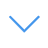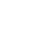# Maths Power

Are you sensitive to mathematical calculations? This game challenges the player's responsiveness and mathematical computing skills. This is a game that has 10 stages, wherein you have to choose the numbers to get the required end result. The game starts off simply with additions and subtractions but remains fairly easy to complete. when you reach the 10th stage, there are more calculated.

Read Moreاضغط على 【Esc】 للخروج من وضع ملء الشاشة

# Maths Power

Are you sensitive to mathematical calculations? This game challenges the player's responsiveness and mathematical computing skills. This is a game that has 10 stages, wherein you have to choose the numbers to get the required end result. The game starts off simply with additions and subtractions but remains fairly easy to complete. when you reach the 10th stage, there are more calculated.لتشغيل اللعبة ، تحتاج إلى السماح بتشغيل Adobe Flash Player في متصفحك.

You can use UGameZone Flash Enabler plugin to enable Flash automatically. Or you can follow our instructions to enable Flash manually.

play automatically

## Game Ratings

82%

يلعب 1130

تقييم 82%

الأصوات 777

777
154
Category
Math Games
الكلمات
وصف

Are you sensitive to mathematical calculations? This game challenges the player's responsiveness and mathematical computing skills. This is a game that has 10 stages, wherein you have to choose the numbers to get the required end result. The game starts off simply with additions and subtractions but remains fairly easy to complete. when you reach the 10th stage, there are more calculated.

Step 1
Step 2